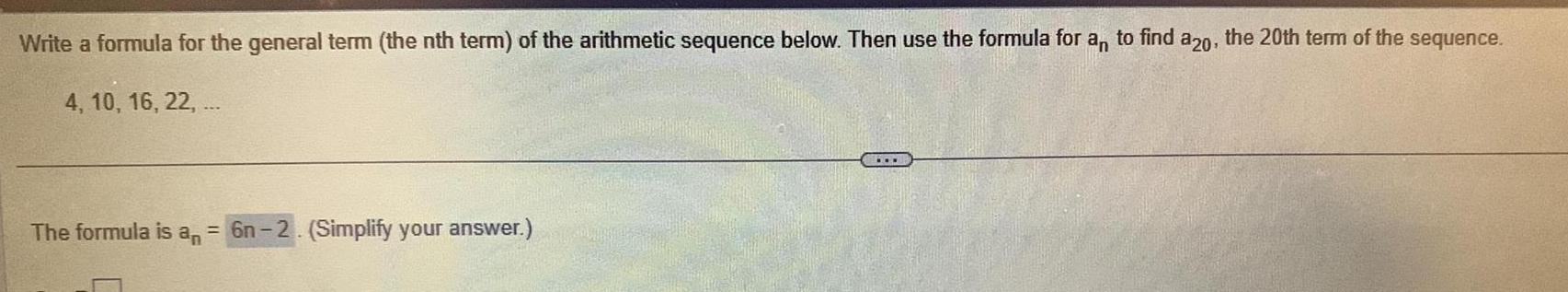Question:

# Write a formula for the general term the nth term of the

Last updated: 11/16/2023Write a formula for the general term the nth term of the arithmetic sequence below Then use the formula for an to find a20 the 20th term of the sequence 4 10 16 22 The formula is an 6n 2 Simplify your answer